• Slides: 15Solving Quadratic Equations What does x = ? ? ?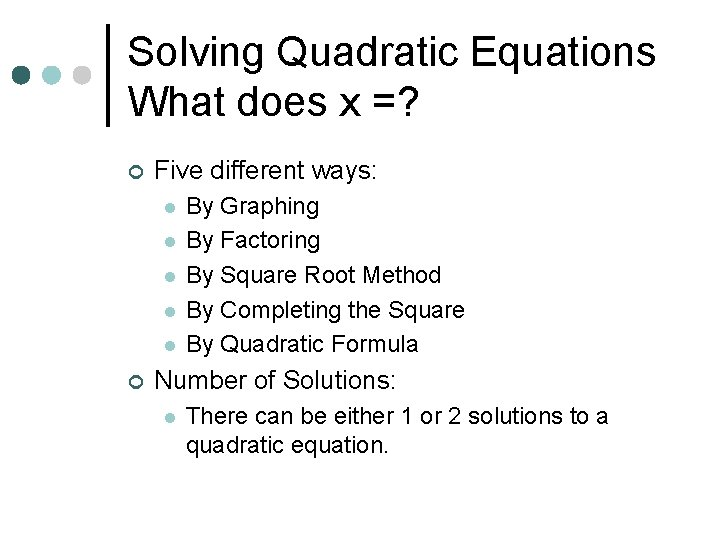Solving Quadratic Equations What does x =? ¢ Five different ways: l l l ¢ By Graphing By Factoring By Square Root Method By Completing the Square By Quadratic Formula Number of Solutions: l There can be either 1 or 2 solutions to a quadratic equation.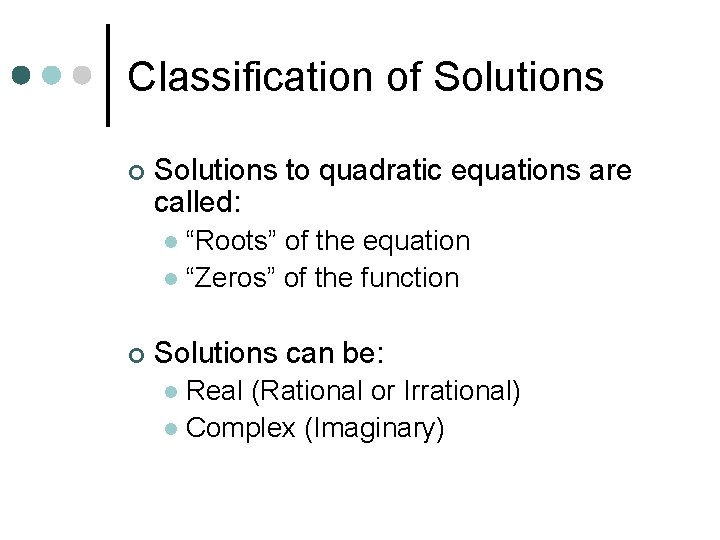Classification of Solutions ¢ Solutions to quadratic equations are called: “Roots” of the equation l “Zeros” of the function l ¢ Solutions can be: Real (Rational or Irrational) l Complex (Imaginary) lClassifying Solutions must be in simplified radical form ¢ If no radicals left, answers are rational. ¢ If radical left, answers are irrational. ¢ Watch out! ¢ l If taking the square root of a negative number, answers are complex (imaginary)!!!!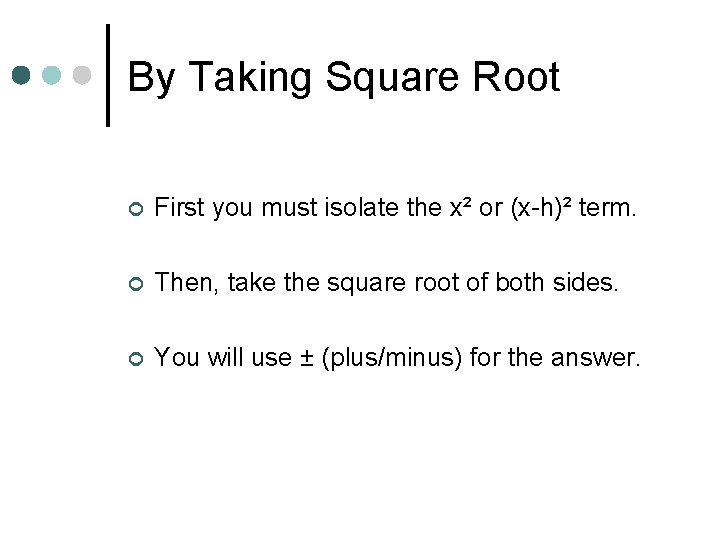By Taking Square Root ¢ First you must isolate the x² or (x-h)² term. ¢ Then, take the square root of both sides. ¢ You will use ± (plus/minus) for the answer.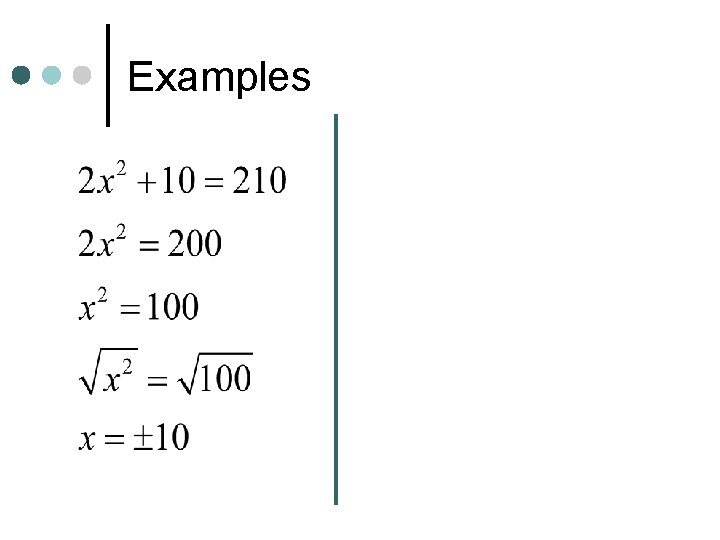ExamplesExamples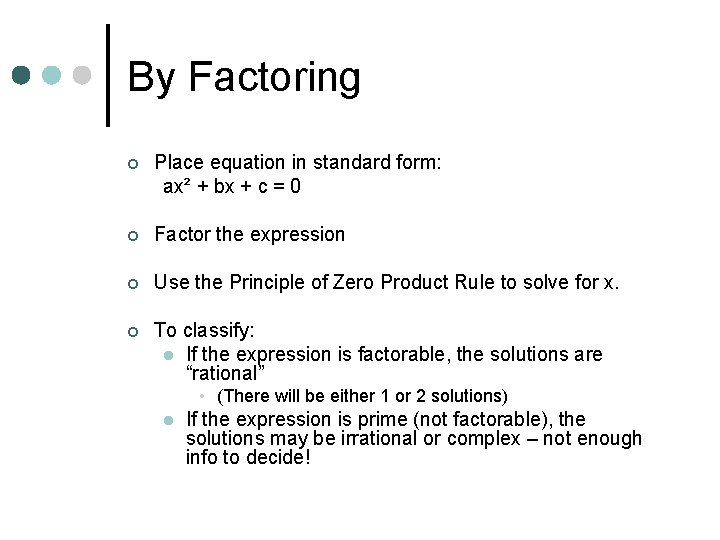By Factoring ¢ Place equation in standard form: ax² + bx + c = 0 ¢ Factor the expression ¢ Use the Principle of Zero Product Rule to solve for x. ¢ To classify: l If the expression is factorable, the solutions are “rational” • (There will be either 1 or 2 solutions) l If the expression is prime (not factorable), the solutions may be irrational or complex – not enough info to decide!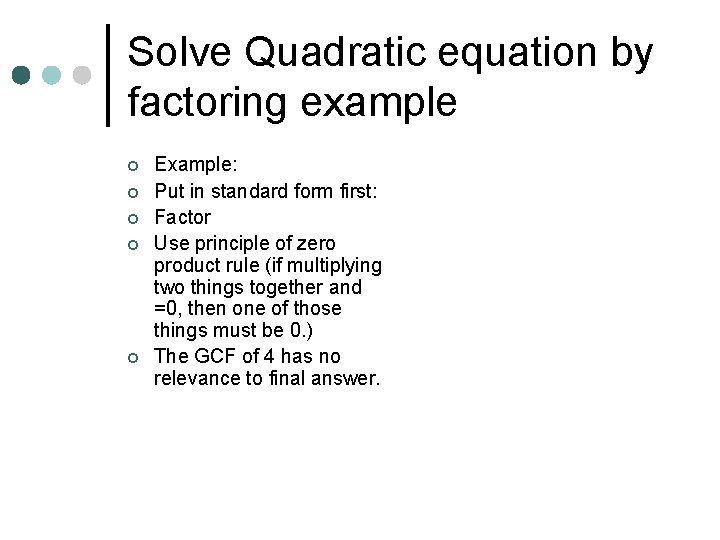Solve Quadratic equation by factoring example ¢ ¢ ¢ Example: Put in standard form first: Factor Use principle of zero product rule (if multiplying two things together and =0, then one of those things must be 0. ) The GCF of 4 has no relevance to final answer.By Completing the Square ¢ Complete the square, then isolate the (x-h)² term. ¢ Solve by square root method.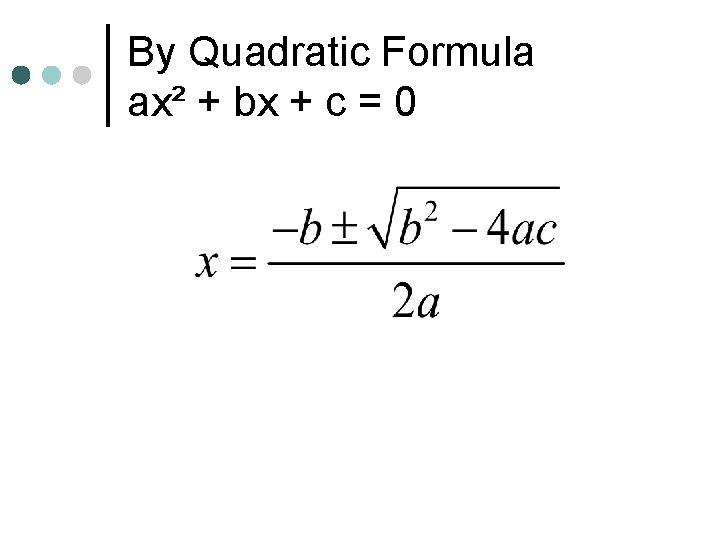By Quadratic Formula ax² + bx + c = 0By Graphing ¢ You have done this! Graph one side of equation in Y 1, other side in Y 2. ¢ 2 nd Calc Intersect to find the intersection of the two functions. ¢ Classify solutions: l If graphs intersect twice, there are 2 solutions. (2 real solutions) ¢ l If graphs intersect once, there is 1 solution (1 real solution) l If graphs never intersect, there are no “real” solutions, but there are 2 complex solutionsDiscriminant-used to classify solutions of quadratic equations ¢ The discriminant is the radicand portion of the quadratic formula: Discriminant = b²-4 ac If discriminant = 0, one rational solution If discriminant = perfect square number, 2 rational solutions If discriminant = non-perfect square number, 2 irrational solutions If discriminant = negative number, 2 complex solutions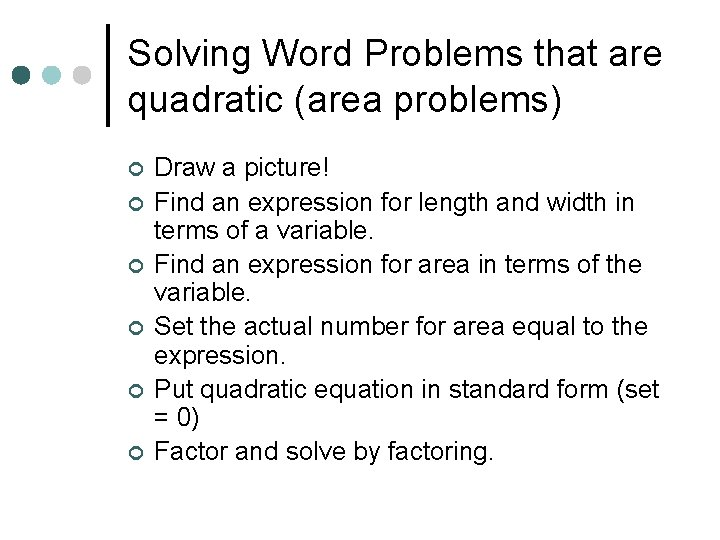Solving Word Problems that are quadratic (area problems) ¢ ¢ ¢ Draw a picture! Find an expression for length and width in terms of a variable. Find an expression for area in terms of the variable. Set the actual number for area equal to the expression. Put quadratic equation in standard form (set = 0) Factor and solve by factoring.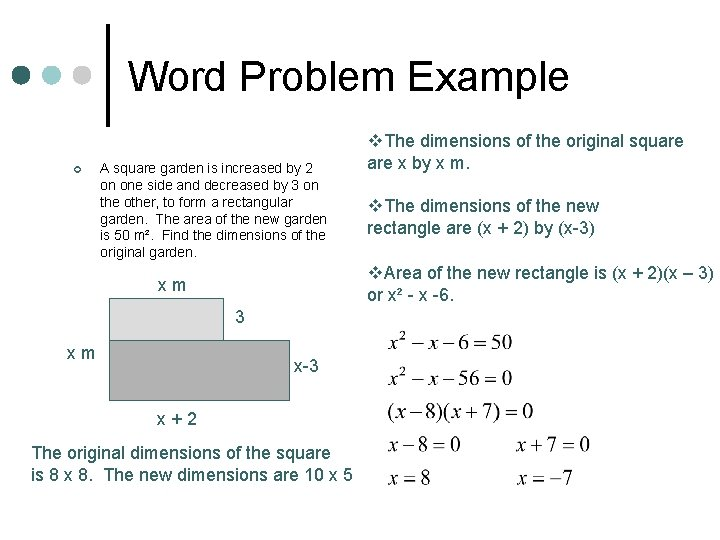Word Problem Example ¢ A square garden is increased by 2 on one side and decreased by 3 on the other, to form a rectangular garden. The area of the new garden is 50 m². Find the dimensions of the original garden. v. The dimensions of the new rectangle are (x + 2) by (x-3) v. Area of the new rectangle is (x + 2)(x – 3) or x² - x -6. xm 3 xm v. The dimensions of the original square x by x m. x-3 x+2 The original dimensions of the square is 8 x 8. The new dimensions are 10 x 5